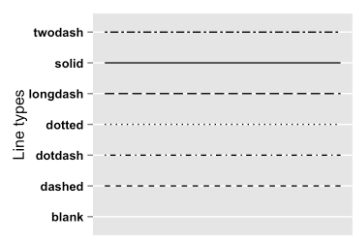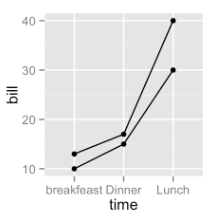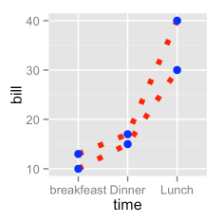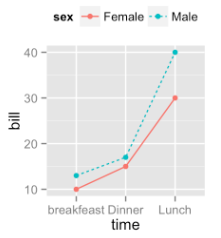R绘图 第六篇：绘制线图（ggplot2）

geom_line(mapping = NULL, data = NULL, stat = "identity",
position = "identity", na.rm = FALSE, show.legend = NA,
inherit.aes = TRUE, ...)

线图中的常用参数：

• group：线的分组
• alpha：线的透明度
• color：线的颜色
• size：线的粗细
• linetype：线的类型，R中可用的类型如下图所示：df <- data.frame(sex = rep(c("Female", "Male"), each=3),
time=c("breakfeast", "Lunch", "Dinner"),
bill=c(10, 30, 15, 13, 40, 17) )

一，绘制线图

ggplot(data=df,mapping=aes(x=time,y=bill,group=sex))+
geom_line()+
geom_point()二，修改线图的线形，颜色和大小

ggplot(data=df, mapping=aes(x=time, y=bill, group=sex)) +
geom_line(linetype="dotted", color="red", size=2)+
geom_point(color="blue", size=3)三，对不同的线图使用不同的颜色

ggplot(data=df, mapping=aes(x=time, y=bill, group=sex)) +
geom_line(aes(linetype=sex,color=sex))+
geom_point(aes(color=sex))+
theme(legend.position="top")四，自定义各个分组的线形、颜色和大小

• scale_linetype_manual() ：控制线形
• scale_color_manual() ：控制线的颜色
• scale_size_manual() ：控制线的粗细

ggplot(df, aes(x=time, y=bill, group=sex)) +
geom_line(aes(linetype=sex, color=sex, size=sex))+
geom_point()+
scale_linetype_manual(values=c("twodash", "dotted"))+
scale_color_manual(values=c('#999999','#E69F00'))+
scale_size_manual(values=c(1, 1.5))+
theme(legend.position="top")Connect observations

posted @ 2018-08-04 09:49  悦光阴  阅读(30931)  评论(0编辑  收藏  举报